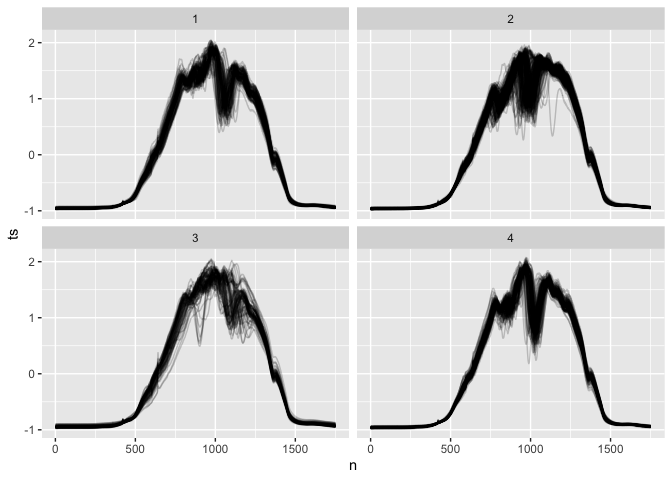The goal of tsrecipes is to provide time series proprocessing to accommodate time series classification and clustering in the tidymodels framework.

The primary steps are

• discrete cosine transform for dimensionality reduction and feature engineering
• dynamic time warping clustering

## Installation

You can install tsrecipes with:

# install.packages("devtools")
devtools::install_github("tmastny/tsrecipes")

## Examples

### Time series dimensionality reduction

In time series classification, using the time series as a feature often results in poor accuracy because it’s the auto-correlation between entries and the overall trend that’s the most informative, rather than individual values.

The discrete cosine transform is one way to extract useful, uncorrelated features with significantly fewer dimensions than the time series.

In this example, it’s possible to classify time series of length 1751 into 4 classes with 70% accuracy, only using 16 dimensions.

library(tidymodels)
library(tsrecipes)

rec <- recipe(ethanol, var = names(ethanol), roles = c("id", "outcome", "input")) %>%
step_dct(ts, k = tune())

set.seed(2532)
tune_results <- workflow() %>%
tune_grid(
resamples = validation_split(ethanol),
grid = expand_grid(k = c(4, 8, 16, 32, 64))
)

tune_results %>%
collect_metrics() %>%
filter(.metric == "accuracy") %>%
select(k, mean)
#> # A tibble: 5 x 2
#>       k  mean
#>   <dbl> <dbl>
#> 1     4 0.278
#> 2     8 0.389
#> 3    16 0.754
#> 4    32 0.746
#> 5    64 0.849

### Time series clustering

tsrecipes also enables times series clustering using the dynamic time warping metric. step_dtw is powered by the dtwclust package.

ethanol_clusters <- recipe(ethanol) %>%
step_dtw(ts, k = 4) %>%
prep() %>%
bake(ethanol)
ethanol_clusters %>%
mutate(n = list(1:1751)) %>%
unnest(c(ts, n)) %>%
ggplot() +
geom_line(aes(n, ts, group = id), alpha = 0.2, show.legend = FALSE) +
facet_wrap(~dtwclust_ts)